# 对Java静态数组的二次封装

## 数组的特点

• 数组最大的优点是可以快速查询，因为数组直接通过索引查询很快：array，所以在随机访问时有一定的优势。其数据结构是简单的线性序列，这使得元素访问非常快速，并且按照索引遍历数组方便。
• 数组最好应用于“索引有语意”的情况
• 但并非所有有语意的索引都适用于数组，例如索引是身份证号这种长度的数字，就无法作为索引使用
• 而数组也同样可以处理“索引没有语意”的情况
• 数组的缺点：
– 根据内容查找元素速度慢
– 数组的大小一经确定不能改变
– 数组只能存储一种类型的数据
– 插入、指定删除元素效率低
– 未封装任何方法，所有操作都需要用户自己定义

## 讲台数组二次封装

• 索引没有语意，如何表示没有元素？
• 如何添加元素？如何删除元素？如何修改元素？

### 主体框架

``public class Array {    /** * 实际存放数据的数组 */    private int[] data;    /** * 表示数组中元素的个数 */    private int size;    /** * 传入数组的容量capacity构造Array * 以便用户自定义数组的容量 * * @param capacity 容量 */    public Array(int capacity) {        data = new int[capacity];        size = 0;    }    public Array() {        // 默认数组容量为10        this(10);    }    /** * 获取数组中元素的个数 * * @return 数组中元素的个数 */    public int getSize() {        return size;    }    /** * 获取数组的容量 * * @return 数组的容量 */    public int getCapacity() {        return data.length;    }    /** * 返回数组是否为空 * * @return 为空返回true，否则返回false */    public boolean isEmpty() {        return size == 0;    }    @Override    public String toString() {        if (isEmpty()) {            return "[]";        }        StringBuilder sb = new StringBuilder();        sb.append(String.format("Array: size = %d, capacity = %d\n", size, data.length));        sb.append("[");        for (int i = 0; i < size; i++) {            sb.append(data[i]);            if (i != size - 1) {                sb.append(", ");            }        }        return sb.append("]").toString();    }}``

### 向数组中添加元素

``/** * 向最后一个元素+1的位置添加一个新的元素 * * @param e 新的元素 */public void addLast(int e) {    // 若数组已满则抛出异常，这里暂时先不做动态扩容    if (size == data.length) {        throw new IllegalArgumentException("AddLast failed. Array is full.");    }    data[size] = e;    size++;}``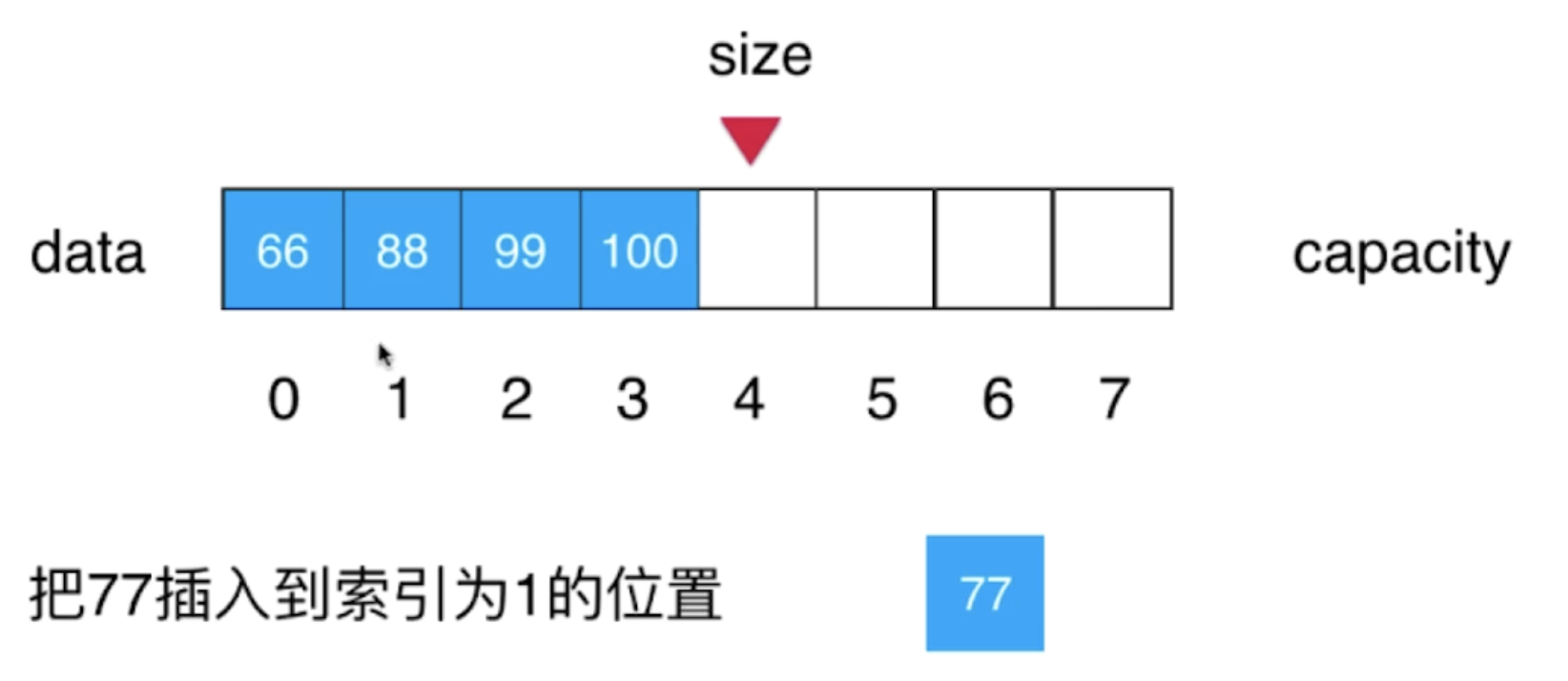``/** * 在第index的位置下插入一个新的元素e * * @param index 索引 * @param e     新的元素 */public void add(int index, int e) {    if (size == data.length) {        throw new IllegalArgumentException("Add failed. Array is full.");    }    // 若索引为负数或索引大于size，则抛出异常    // 因为index大于size会造成数组内的元素不具有连续性，而index小于0则是无效的索引    if (index < 0 || index > size) {        throw new IllegalArgumentException("Add failed. Require index >= 0 and index <= size.");    }    // 从最后一个元素开始遍历数组中的元素，直到抵达index指向的索引位置    for (int i = size - 1; i >= index; i--) {        // 每个元素向后挪一位        data[i + 1] = data[i];    }    // 也可以直接使用数组拷贝的函数来实现这个逻辑，这里为了表明这个插入的逻辑，所以没有使用数组拷贝    // System.arraycopy(data, index, data, index + 1, size - index);      // 在index的位置插入新的元素    data[index] = e;    // 最后size需+1    size++;}``

``/** * 向最后一个元素+1的位置添加一个新的元素 * * @param e 新的元素 */public void addLast(int e) {    add(size, e);}``

``/** * 往所有元素前添加一个新的元素 * * @param e 新的元素 */public void addFirst(int e) {    add(0, e);}``

### 数组中查询元素和修改元素

``/** * 检查索引是否合法 * * @param index index */private void checkIndex(int index) {    // 若索引为负数或索引大于等于size，则抛出异常    // 因为index大于等于size时用户会访问到无效的数据，而index小于0则是无效的索引    if (index < 0 || index >= size) {        throw new IllegalArgumentException("Require index >= 0 and index <= size.");    }}``

``/** * 获取index索引位置的元素 * * @param index index * @return index索引位置的元素 */public int get(int index) {    checkIndex(index);    return data[index];}/** * 获取数组中的最后一个元素 * * @return 最后一个元素 */public int getLast() {    return get(size - 1);}/** * 获取数组中的第一个元素 * * @return 第一个元素 */public int getFirst() {    return get(0);}/** * 修改index索引位置的元素为e * * @param index index * @param e     e */public void set(int index, int e) {    checkIndex(index);    data[index] = e;}``

### 包含，搜索和删除

``/** * 查找数组中是否有元素e * * @param e e * @return 有返回true，没有返回false */public boolean contains(int e) {    int index = indexOf(e);    return index != -1;}/** * 查找数组中元素e所在的索引，如果不存在元素e，则返回-1 * * @param e e * @return 元素e所在的索引，或-1 */public int indexOf(int e) {    for (int i = 0; i < size; i++) {        if (data[i] == e) {            return i;        }    }    return -1;}``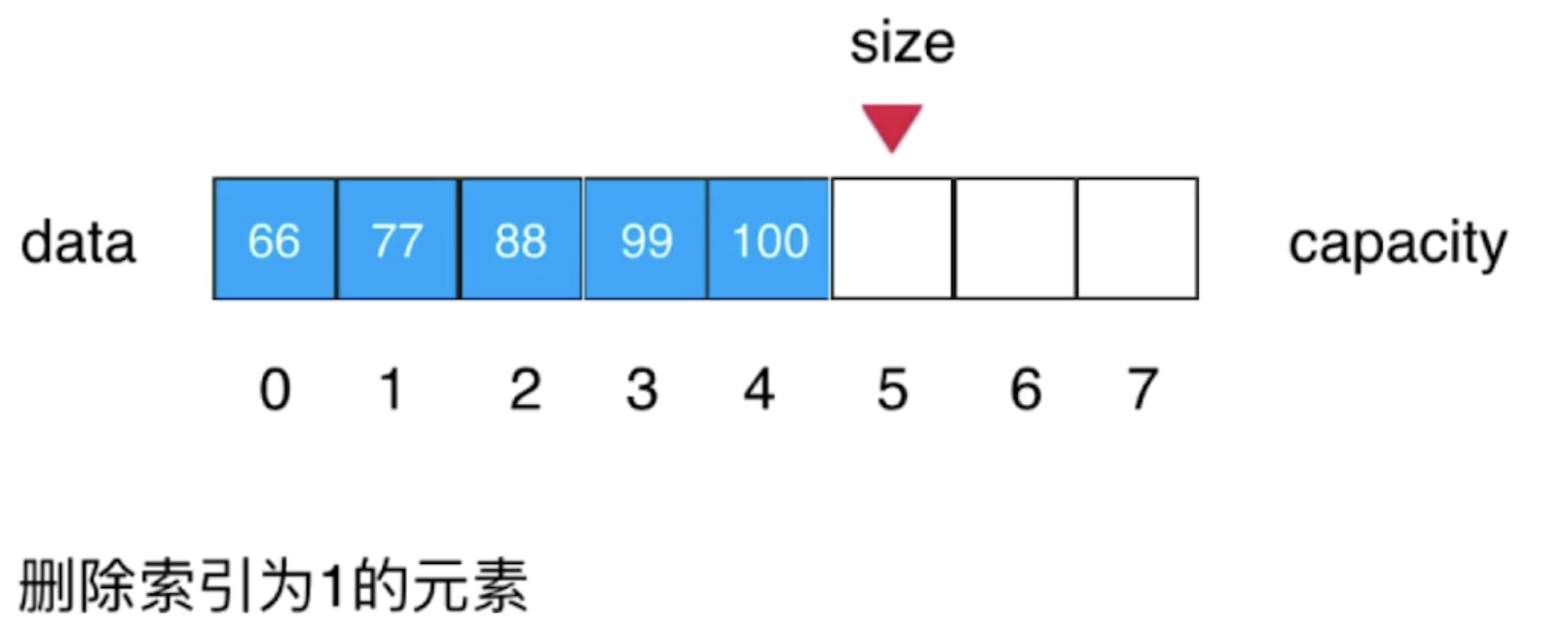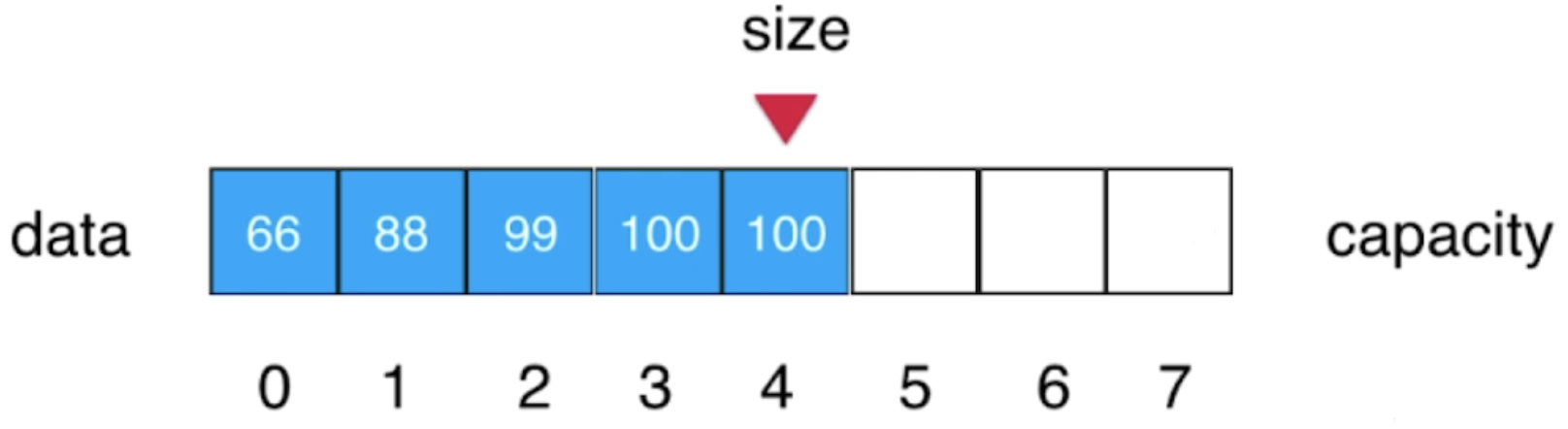``/** * 从数组中删除index位置的元素，并返回删除的元素 * * @param index index * @return 被删除的元素 */public int remove(int index) {    checkIndex(index);    int ret = data[index];    for (int i = index + 1; i < size; i++) {        data[i - 1] = data[i];    }    // 同样的，这里也可以直接使用数组拷贝的函数来实现这个逻辑    // System.arraycopy(data, index + 1, data, index, size - index + 1);    size--;    data[size] = 0;    return ret;}``

``/** * 从数组中删除第一个元素，并返回删除的元素 * * @return 被删除的元素 */public int removeFirst() {    return remove(0);}/** * 从数组中删除最后一个元素，并返回删除的元素 * * @return 被删除的元素 */public int removeLast() {    return remove(size - 1);}/** * 从数组中删除元素e * * @param e 需要删除的元素 * @return 删除成功返回true，否则返回false */public boolean removeElement(int e) {    int index = indexOf(e);    if (index != -1) {        remove(index);        return true;    }    return false;}/** * 删除所有的元素e * * @param e 需要删除的元素 * @return 删除成功返回true，否则返回false */public boolean removeAllElement(E e) {    for (int i = 0; i < size; ) {        if (data[i] == e) {            remove(i);        } else {            i++;        }    }    return indexOf(e) == -1;}``

### 使用泛型

``public class Array<E> {    /**     * 实际存放数据的数组     */    private E[] data;    /**     * 数组中元素的个数     */    private int size;    /**     * 传入数组的容量capacity构造Array     * 以便用户自定义数组的容量     *     * @param capacity 容量     */    public Array(int capacity) {        // java语法不支持直接new泛型或泛型数组，所以我们需要先new一个Object进行强转        data = (E[]) new Object[capacity];        size = 0;    }    public Array() {        // 默认数组容量为10        this(10);    }    /**     * 获取数组中元素的个数     *     * @return 数组中元素的个数     */    public int getSize() {        return size;    }    /**     * 获取数组的容量     *     * @return 数组的容量     */    public int getCapacity() {        return data.length;    }    /**     * 返回数组是否为空     *     * @return 为空返回true，否则返回false     */    public boolean isEmpty() {        return size == 0;    }    /**     * 向最后一个元素+1的位置添加一个新的元素     *     * @param e 新的元素     */    public void addLast(E e) {        add(size, e);    }    /**     * 往所有元素前添加一个新的元素     *     * @param e 新的元素     */    public void addFirst(E e) {        add(0, e);    }    /**     * 在第index的位置下插入一个新的元素e     *     * @param index 索引     * @param e     新的元素     */    public void add(int index, E e) {        // 若数组已满则抛出异常        if (size == data.length) {            throw new IllegalArgumentException("Add failed. Array is full.");        }        // 若索引为负数或索引大于size，则抛出异常        // 因为index大于size会造成数组内的元素不具有连续性，而index小于0则是无效的索引        if (index < 0 || index > size) {            throw new IllegalArgumentException("Add failed. Require index >= 0 and index <= size.");        }        // 从最后一个元素开始遍历数组中的元素，直到抵达index指向的索引位置        for (int i = size - 1; i >= index; i--) {            // 每个元素向后挪一位            data[i + 1] = data[i];        }        // 也可以直接使用数组拷贝的函数来实现这个逻辑，这里为了表明这个插入的逻辑，所以没有使用数组拷贝        // System.arraycopy(data, index, data, index + 1, size - index);        data[index] = e;        size++;    }    /**     * 获取index索引位置的元素     *     * @param index index     * @return index索引位置的元素     */    public E get(int index) {        checkIndex(index);        return data[index];    }    /**     * 获取数组中的最后一个元素     *     * @return 最后一个元素     */    public E getLast() {        return get(size - 1);    }    /**     * 获取数组中的第一个元素     *     * @return 第一个元素     */    public E getFirst() {        return get(0);    }    /**     * 修改index索引位置的元素为e     *     * @param index index     * @param e     e     */    public void set(int index, E e) {        checkIndex(index);        data[index] = e;    }    /**     * 查找数组中是否有元素e     *     * @param e e     * @return 有返回true，没有返回false     */    public boolean contains(E e) {        int index = indexOf(e);        return index != -1;    }    /**     * 查找数组中元素e所在的索引，如果不存在元素e，则返回-1     *     * @param e e     * @return 元素e所在的索引，或-1     */    public int indexOf(E e) {        for (int i = 0; i < size; i++) {            if (data[i].equals(e)) {                return i;            }        }        return -1;    }    /**     * 从数组中删除index位置的元素，并返回删除的元素     *     * @param index index     * @return 被删除的元素     */    public E remove(int index) {        checkIndex(index);        E ret = data[index];        for (int i = index + 1; i < size; i++) {            data[i - 1] = data[i];        }        // 同样的，这里也可以直接使用数组拷贝的函数来实现这个逻辑        // System.arraycopy(data, index + 1, data, index, size - index + 1);        size--;        data[size] = null;        return ret;    }    /**     * 从数组中删除第一个元素，并返回删除的元素     *     * @return 被删除的元素     */    public E removeFirst() {        return remove(0);    }    /**     * 从数组中删除最后一个元素，并返回删除的元素     *     * @return 被删除的元素     */    public E removeLast() {        return remove(size - 1);    }    /**     * 从数组中删除元素e     *     * @param e 需要删除的元素     * @return 删除成功返回true，否则返回false     */    public boolean removeElement(E e) {        int index = indexOf(e);        if (index != -1) {            remove(index);            return true;        }        return false;    }    /**     * 删除所有的元素e     *     * @param e 需要删除的元素     * @return 删除成功返回true，否则返回false     */    public boolean removeAllElement(E e) {        for (int i = 0; i < size; ) {            if (data[i] == e) {                remove(i);            } else {                i++;            }        }        return indexOf(e) == -1;    }    @Override    public String toString() {        if (isEmpty()) {            return "[]";        }        StringBuilder sb = new StringBuilder();        sb.append(String.format("Array: size = %d, capacity = %d\n", size, data.length));        sb.append("[");        for (int i = 0; i < size; i++) {            sb.append(data[i]);            if (i != size - 1) {                sb.append(", ");            }        }        return sb.append("]").toString();    }    /**     * 检查索引是否合法     *     * @param index index     */    private void checkIndex(int index) {        // 若索引为负数或索引大于等于size，则抛出异常        // 因为index大于等于size时用户会访问到无效的数据，而index小于0则是无效的索引        if (index < 0 || index >= size) {            throw new IllegalArgumentException("Require index >= 0 and index <= size.");        }    }}``

### 动态数组

``/** * 重置数组容量 * * @param newCapacity 新的容量 */private void resize(int newCapacity) {    E[] newData = (E[]) new Object[newCapacity];    for (int i = 0; i < size; i++) {        newData[i] = data[i];    }    // 可以直接使用数组拷贝的方式    // System.arraycopy(data, 0, newData, 0, size);    data = newData;}``

``/** * 在第index的位置下插入一个新的元素e * * @param index 索引 * @param e     新的元素 */public void add(int index, E e) {    // 若索引为负数或索引大于size，则抛出异常    // 因为index大于size会造成数组内的元素不具有连续性，而index小于0则是无效的索引    if (index < 0 || index > size) {        throw new IllegalArgumentException("Add failed. Require index >= 0 and index <= size.");    }    // 若数组已满则进行扩容    if (size == data.length) {        resize(data.length * 2);    }    // 从最后一个元素开始遍历数组中的元素，直到抵达index指向的索引位置    for (int i = size - 1; i >= index; i--) {        // 每个元素向后挪一位        data[i + 1] = data[i];    }    // 也可以直接使用数组拷贝的函数来实现这个逻辑，这里为了表明这个插入的逻辑，所以没有使用数组拷贝    // System.arraycopy(data, index, data, index + 1, size - index);    data[index] = e;    size++;}``

``/** * 从数组中删除index位置的元素，并返回删除的元素 * * @param index index * @return 被删除的元素 */public E remove(int index) {    checkIndex(index);    E ret = data[index];    for (int i = index + 1; i < size; i++) {        data[i - 1] = data[i];    }    // 同样的，这里也可以直接使用数组拷贝的函数来实现这个逻辑    // System.arraycopy(data, index + 1, data, index, size - index + 1);    size--;    data[size] = null;    // 判断是否需要缩容，这里4分之1才缩容是为了避免复杂度震荡    if (size == data.length / 4 && data.length / 2 != 0) {        resize(data.length / 2);    }    return ret;}``

### 时间复杂度比较

set(index, e)：这种根据索引修改元素的复杂度显然是O(1)，因为通过索引可以直接访问/操作数组元素

get(index)：同样的，该方法是根据索引直接获取元素，复杂度是O(1)

contains(e)：在不知道索引的情况下，我们只能通过遍历数组元素的方式去判断数组中是否包含某个元素，所以复杂度是O(n)

indexOf(e)：查询元素所在的索引也是同样的，最坏的情况需要遍历整个数组，复杂度是O(n)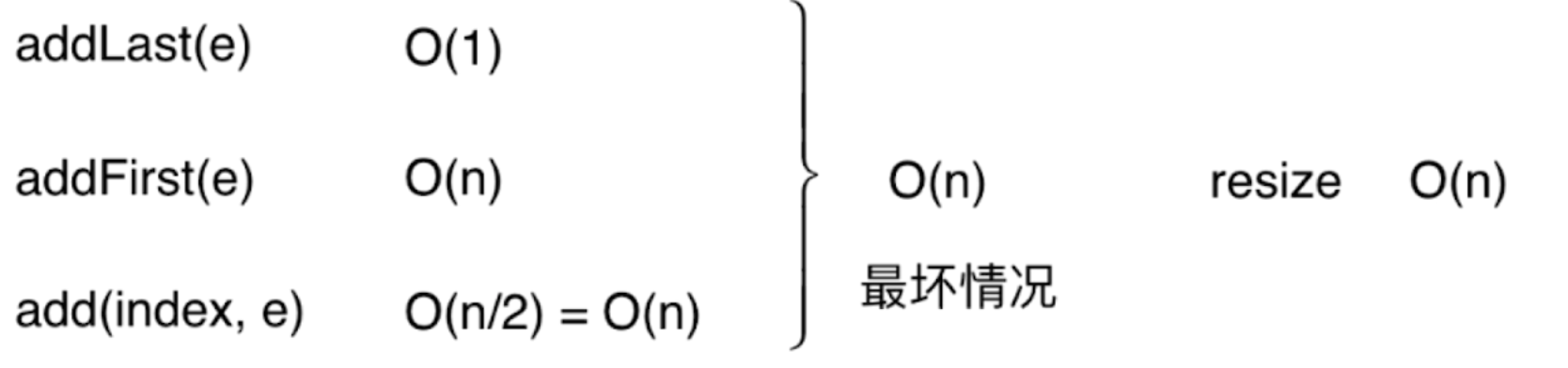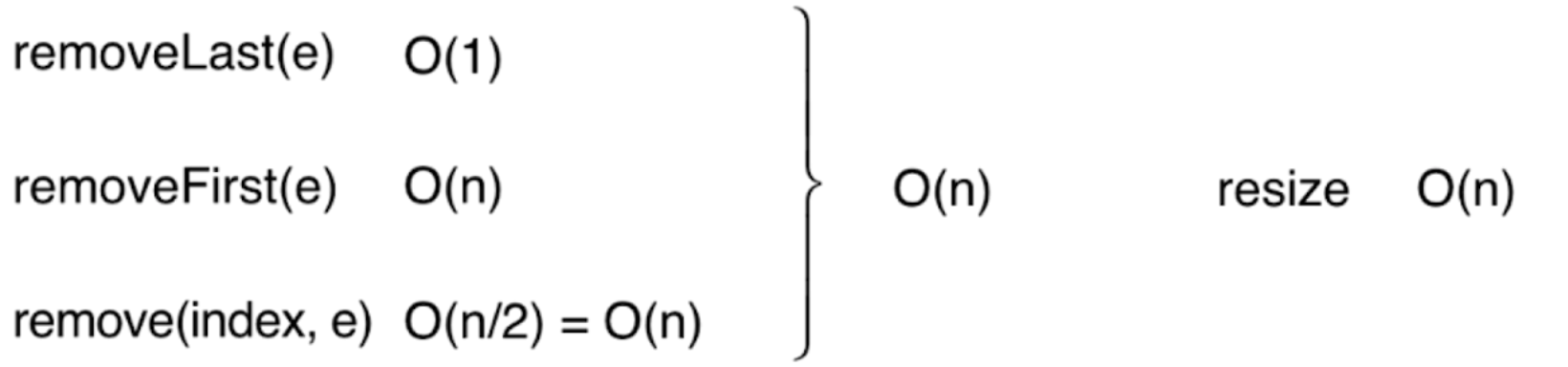• 增：O(n)
• 删：O(n)
• 改：已知索引 O(1)；未知索引 O(n)
• 查：已知索引 O(1)；未知索引 O(n)

##### 热门推荐
•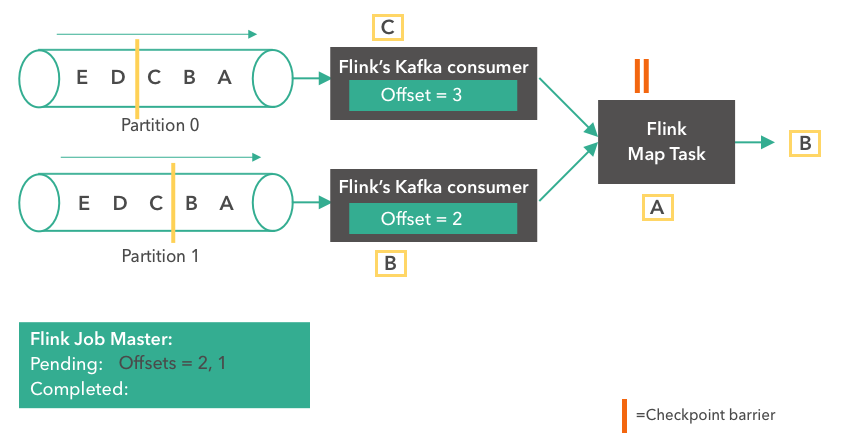浏览(2,357)
•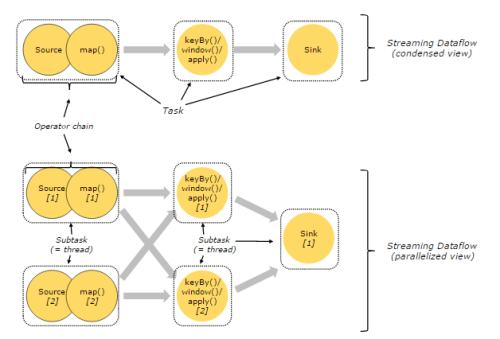浏览(1,659)
•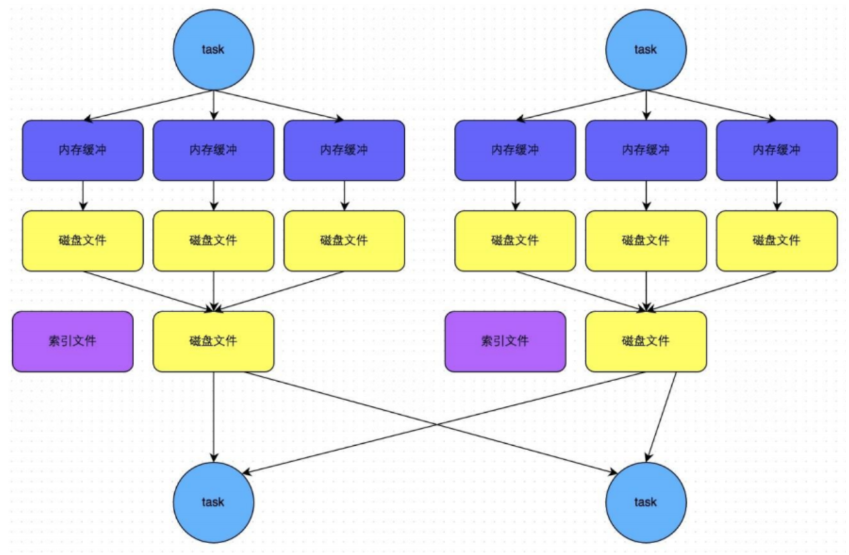浏览(1,646)
•浏览(1,568)
•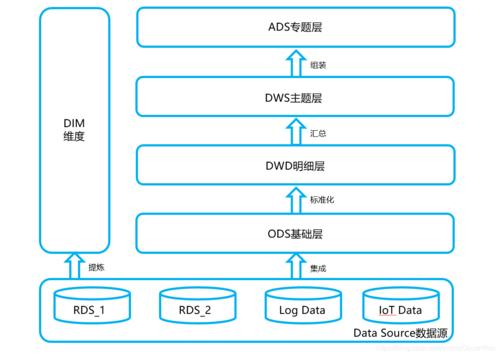浏览(1,562)
•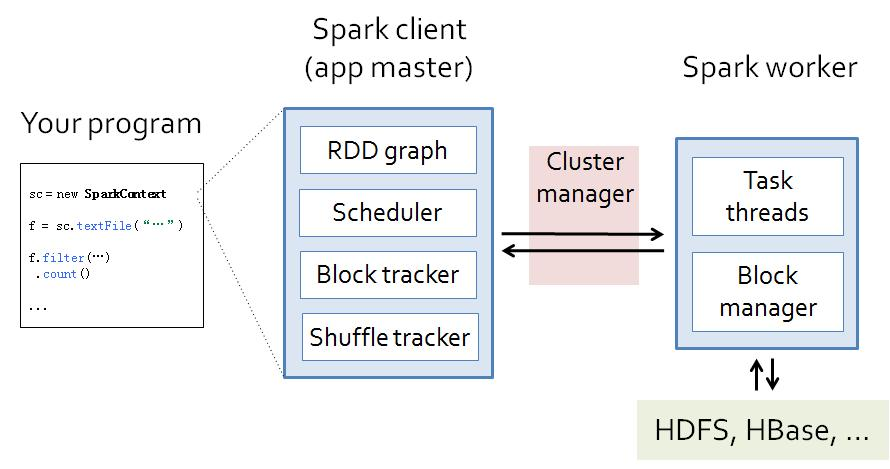浏览(1,539)
•浏览(1,499)
•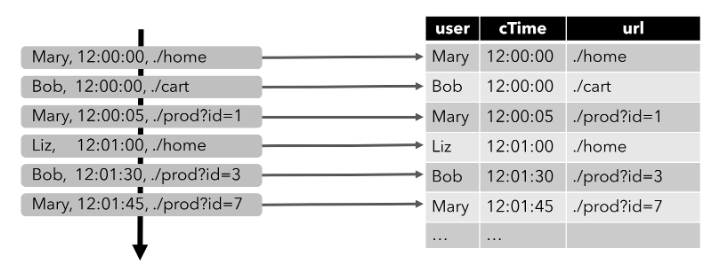浏览(1,437)
•浏览(1,429)
•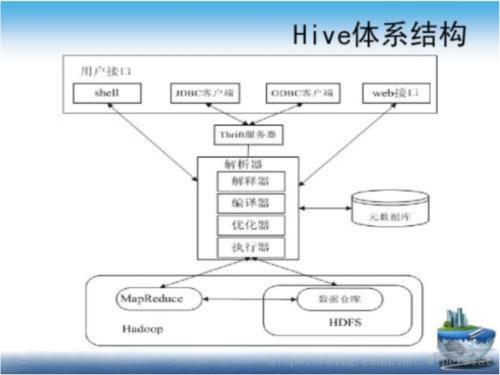浏览(1,399)